### Home > CALC > Chapter 8 > Lesson 8.2.3 > Problem8-95

8-95.
1.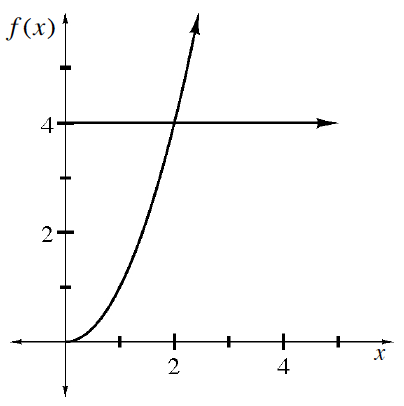Multiple Choice: The function f(x) = x2 is bounded by the y-axis and the line y = 4. The volume generated by revolving the region about the y-axis can be found by which of the following integrals? Homework Help ✎

1.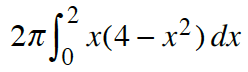2.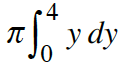3.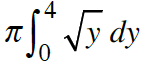2.  a. I only b. II only c. III only d. I and II e. I and III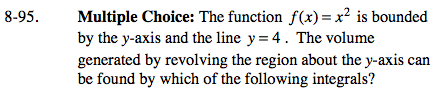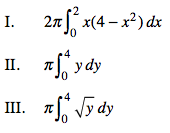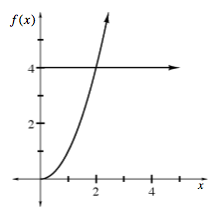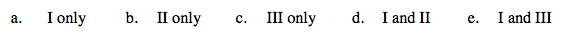If you want to use disks/washers, then rotate horizontal rectangles...
that means that both the bounds and the integrand must be written in terms of y.

$\text{Disks: }\pi \int_{y=a}^{y=b}(f(y))^{2}dy$

$\text{Washers: }\pi \int_{y=a}^{y=b}(f(y))^{2}-(g(y))^{2}dy$

If you want to use shells, then use x-values.